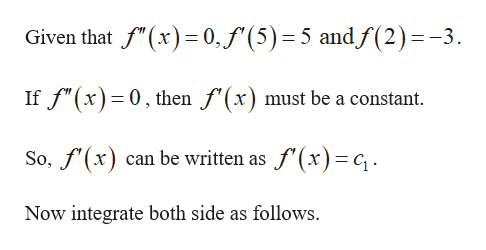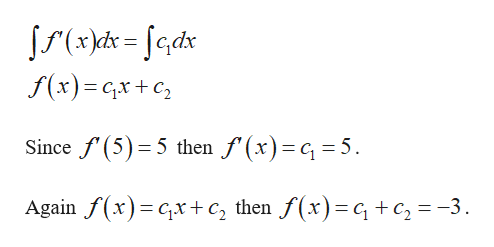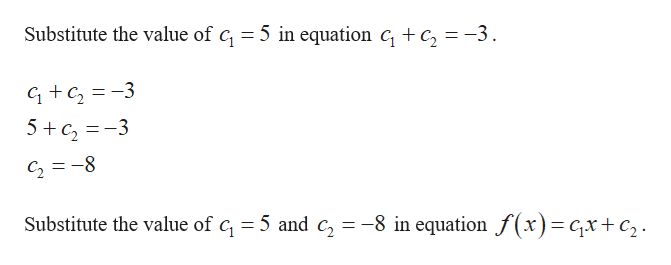# Determine the function f satisfying the given conditions.f '' (x) = 0f ' (5) = 5f (2) = −3f '(x) =  f (x) =

Question
14 views

Determine the function f satisfying the given conditions.

f '' (x) = 0
f ' (5) = 5
f (2) = −3

f '(x) =
f (x) =

check_circle

Step 1help_outlineImage TranscriptioncloseGiven that f"(x) 0,f'(5) 5 andf(2)=-3 If f"(x) 0, then f'(x) must be a constant So,f(x) can be written as f'(x)c Now integrate both side as follows. fullscreen
Step 2help_outlineImage TranscriptioncloseS(xkd=edx f(x) GxC Since f(5) 5 then f'(x)= 5 Again f(x)x+c, then f(x)=q+c 3 fullscreen
Step 3help_outlineImage TranscriptioncloseSubstitute the value of c = 5 in equation c, + c, = -3. GC23 5c3 C28 Substitute the value of c, 5 and c, =-8 in equation f(x)= cx +c2 fullscreen

### Want to see the full answer?

See Solution

#### Want to see this answer and more?

Solutions are written by subject experts who are available 24/7. Questions are typically answered within 1 hour.*

See Solution
*Response times may vary by subject and question.
Tagged in

### Other